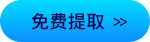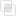Python2021-03-30 10:50:27175浏览 · 0收藏 · 0评论Python判断回文数可以先从控制台输入一个五位数，先判断是否是五位，是否是纯数字组成，如果是回文数就会打印“是回文数”，如果不是就会打印“不是回文数”，下面通过三种方法看下python如何判断回文数。

```a = input('请输入一个五位数：')
# 判断长度是否是5位，是否有纯数字组成if len(a) == 5 and a.isdigit():
# 将str转为inta = int(a)
# （万位与各位） and （千位与十位）
if (a//10000 == a%10) and (a%10000//1000 == a%100 // 10):
print(f'{a}是回文数')else:print(f'{a}不是回文数')else:print('输入有误')```

```a = input('请输入一个五位数：')
# 判断长度是否是5位，是否有纯数字组成
if len(a) == 5 and a.isdigit():
# 对a和a，a和a，进行对比
if (a == a) and (a == a):print('%f是回文数' % (a))else:
print('%f不是回文数' % (a))else:print('输入有误')```

input()函数输入的数据的类型是str，str是有序的，所以可以对str切片操作，并取逆序的结果，将逆序的结果与原来的str做比较

```a = input('请输入一个五位数：')
# 判断长度是否是5位，是否有纯数字组成if len(a) == 5 and a.isdigit():
# 逆序切片的结果与a做比较if a[::-1] == a:print(f'{a}是回文数')else:
print(f'{a}不是回文数')else:print('输入有误')```python2升级到python3防踩坑教程Python、C-Python、Cython代码与GIL的交互使用多线程让Python应用飞起来Python 中拼音库 PyPinyin 的用法Elasticsearch基本介绍及其与Python的对接实现